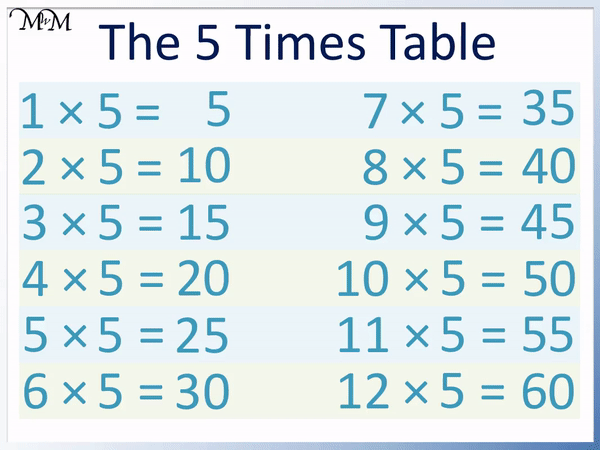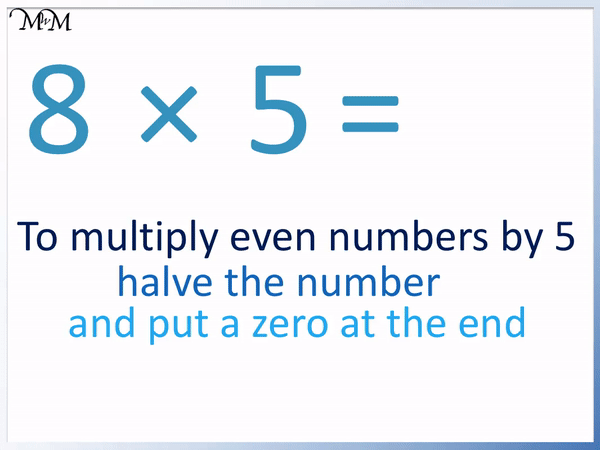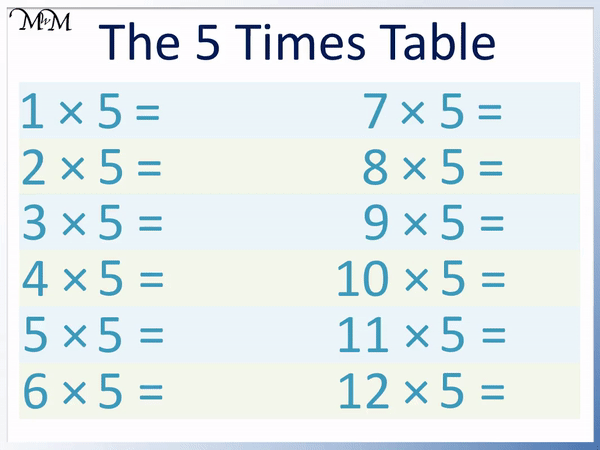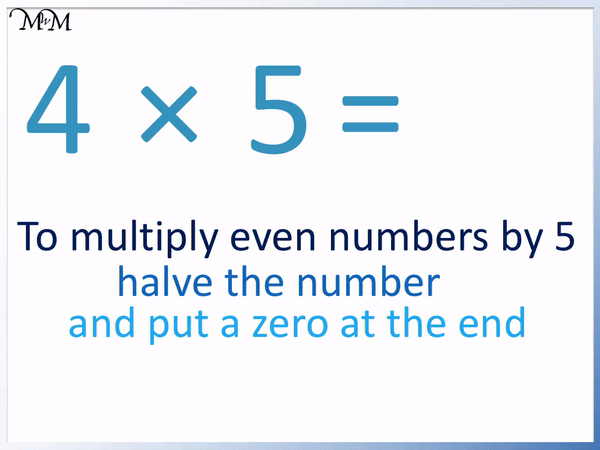# The 5 Times Table

The 5 Times TableThe 5 times table is:

• 1 × 5 = 5
• 2 × 5 = 10.
• 3 × 5 = 15
• 4 × 5 = 20
• 5 × 5 = 25
• 6 × 5 = 30
• 7 × 5 = 35
• 8 × 5 = 40
• 9 × 5 = 45
• 10 × 5 = 50
• 11 × 5 = 55
• 12 × 5 = 60• The five times table is made by counting up in fives.
• The numbers repeat the pattern of ending in 5 and then 0.
• Each ten contains two numbers. One number ending in 0 and one ending in 5.
• To multiply any number by 5, multiply it by ten and halve it.
• To multiply an even number by 5, we can simply halve it and put a zero on the end.
• We can then work out the next number in the five times table by adding a 5 to this number.

To multiply a number by 5, multiply it by ten and then halve it.

The 5 times table repeats the pattern of ending in 0 and then 5.• To multiply an even number by 5, halve the number and put a zero on the end.
• Half of 8 is 4, so we write 4.
• We put a zero on the end of 4 to get 40.
• 8 × 5 = 40.
• This is the same result as multiplying 8 by ten to get 80 and then halving it to get 40.#### 5 Times Table Flashcards

Click on the 5 times table flashcards below to memorise the 5 times table.

×
×

=

# The 5 Times Table

## What is the 5 Times Table?

The 5 times table is formed by counting up in fives:

• 1 × 5 = 5
• 2 × 5 = 10
• 3 × 5 = 15
• 4 × 5 = 20
• 5 × 5 = 25
• 6 × 5 = 30
• 7 × 5 = 35
• 8 × 5 = 40
• 9 × 5 = 45
• 10 × 5 = 50
• 11 × 5 = 55
• 12 × 5 = 60
• Here is the 5 times table chart displaying the full 5 times table.

We can see that each number in the 5 times table can be made by adding 5 to the previous number in the 5 times table.## How to Learn the 5 Times Table

To learn the 5 times table, first notice the repeating pattern of each number ending in 5 and then 0.

Even numbers can be easily multiplied by 5 by halving them and then writing a zero after them. We can simply add 5 to these answers to find any odd numbers multiplied by 5.

This trick works because multiplying by 5 is the same as multiplying by ten and then halving the result. 5 is half of ten.

To multiply any whole number by ten, we can simply write a zero after it.

It is easier to halve the number first and then multiply it by ten.

We can fill in the 5 times table chart below with the even multiples of 5. The trick for learning the 5 times table is to half the even numbers and then put a zero after them.For example here is the multiplication of 4 × 5.

4 is an even number, so we can halve it to get 2.We then put a zero digit on the end of 2 to make 20.

4 × 5 = 20

This is the same result we would get if we multiplied by 10 first and then halved our number.

4 × 10 = 40 and half of 40 is 20.

Here is 6 × 5. Again 6 is an even number and so we can use our 5 times table trick.

We halve 6 to get 3 and then put a zero after 3 to get 30.6 × 5 = 30

We would get this result if we first multiplied by 10 and then halved the answer afterwards.

6 × 10 = 60 and half of 60 is 30.

Here is the example of 8 × 5. Again 8 is even so we can use our 5 times table trick.

Half of 8 is 4 and then putting a zero after 4 gives the answer of 40.8 × 5 = 40

Again, we can multiply 8 by 10 first and then halve it.

8 × 10 = 80 and half of 80 is 40.

The even multiples of 5 are the easiest numbers to learn in the 5 times table.

We can then find all of the rest of the 5 times table by adding 5 on to the numbers that we already know.

Here is the complete 5 times table chart being filled in by adding 5 to the even multiples.For example, if 2 × 5 = 10, then we can easily find 3 × 5 by adding 5 more on to this result.

10 + 5 = 15 and so, 3 × 5 = 15.

If we want to find 9 × 5, then we can first find 8 × 5 and then add 5 to this answer.

8 × 5 = 40 and so 9 × 5 = 45.

Below is the complete 5 times table list.

We can see patterns in the 5 times table that help us to memorise it.We can see that there are two numbers in each tens digit.

There are two numbers with 1 as a tens digit: 10 and 15.

There are two numbers with 2 as a tens digit: 20 and 25.

There are two numbers with 3 as a tens digit: 30 and 35.

There are two numbers with a 4 as a tens digit: 40 and 45.

There are two numbers with a 5 as a tens digit: 50 and 55.

12 × 5 = 60 and if we included 13 × 5, we would have an answer of 13 × 5 = 65.

We would then also have two numbers with a 6 as a tens digit.

We can see that each pair of numbers has a number ending in 0 and the next number ending in 5.

We can work out any number in the 5 times table from the number before it.

If the previous number ends in a 0, then the next number in the 5 times table is the same but the 0 becomes a 5.

If the previous number ends in a 5, then the next number in the 5 times table ends in a 0 and the tens digit is one larger.Now try our lesson on Multiplying by Multiples of 10 and 100 where we learn how to multiply by larger numbers ending in a zero.error: Content is protected !!